# Does Current Flow Through a Capacitor?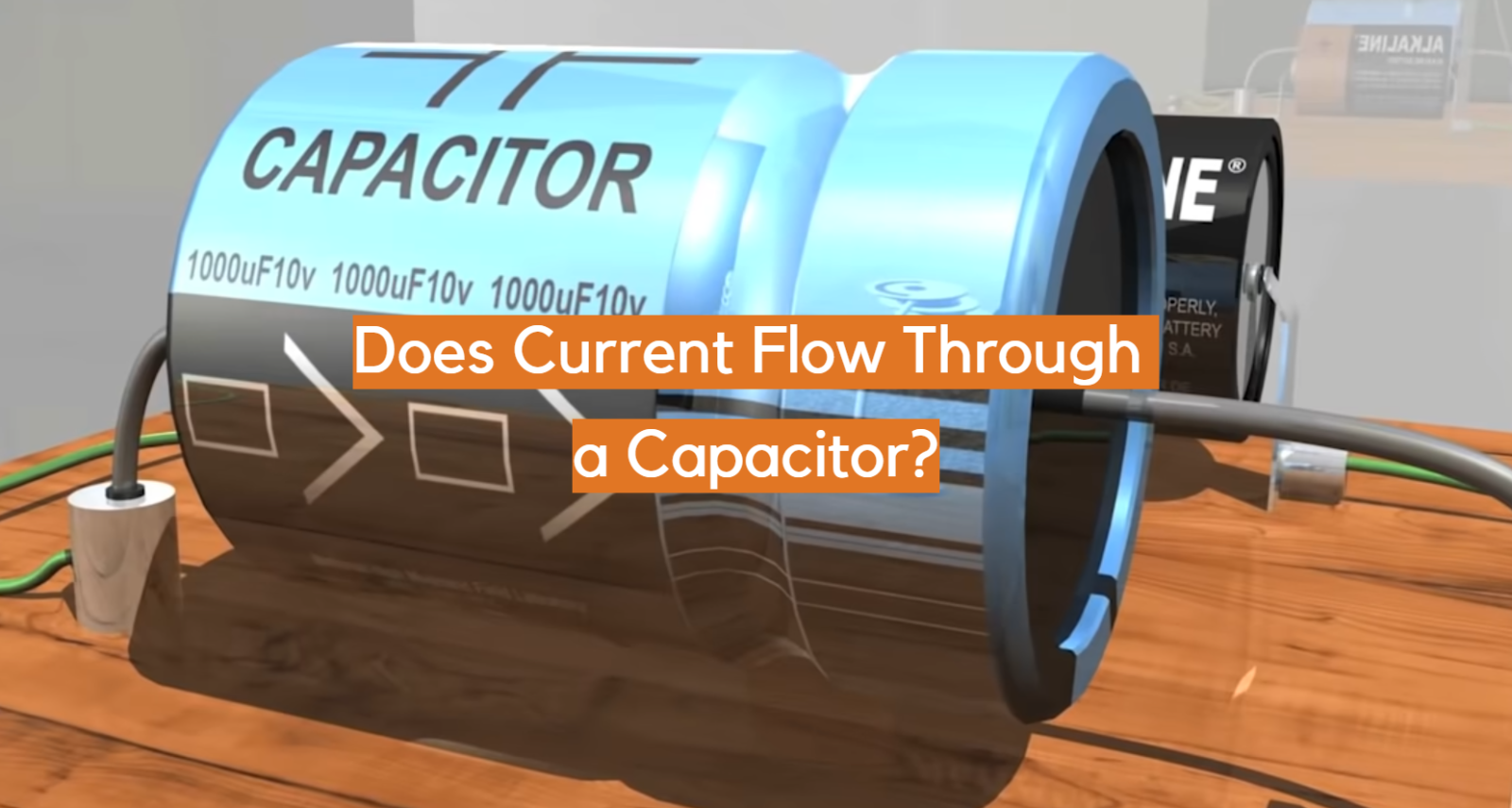When it comes to understanding the behavior of capacitors in electrical circuits, one of the common questions that arise is whether current actually flows through a capacitor. Capacitors are passive components known for their ability to store and release electrical energy. However, their operation can be quite perplexing, especially for those new to the world of electronics. In this article, we will delve into the intriguing concept of current flow in capacitors and explore the factors that influence it.

At first glance, capacitors may seem like insulators due to their ability to store charge. Yet, they are not entirely impervious to the flow of electric current. While it is true that capacitors block direct current (DC), they do allow for the flow of alternating current (AC).

The behavior of current in a capacitor depends on various factors such as the voltage applied, the frequency of the AC signal, and the capacitance of the capacitor itself. By understanding these intricacies, we can gain insight into how capacitors operate in different circuit configurations.

In this article, we will address common questions related to current flow in capacitors. We will explore the behavior of capacitors in both DC and AC circuits, examine the concept of capacitive reactance, discuss the factors that influence current flow, and clarify the circumstances under which current stops flowing through a capacitor. By unraveling the mysteries surrounding current flow in capacitors, we can enhance our understanding of these fundamental components and their applications in various electronic systems.

## About The Current Flow in a Capacitive Circuit

A capacitive circuit is an essential component in various electrical systems, playing a crucial role in storing and releasing electrical energy.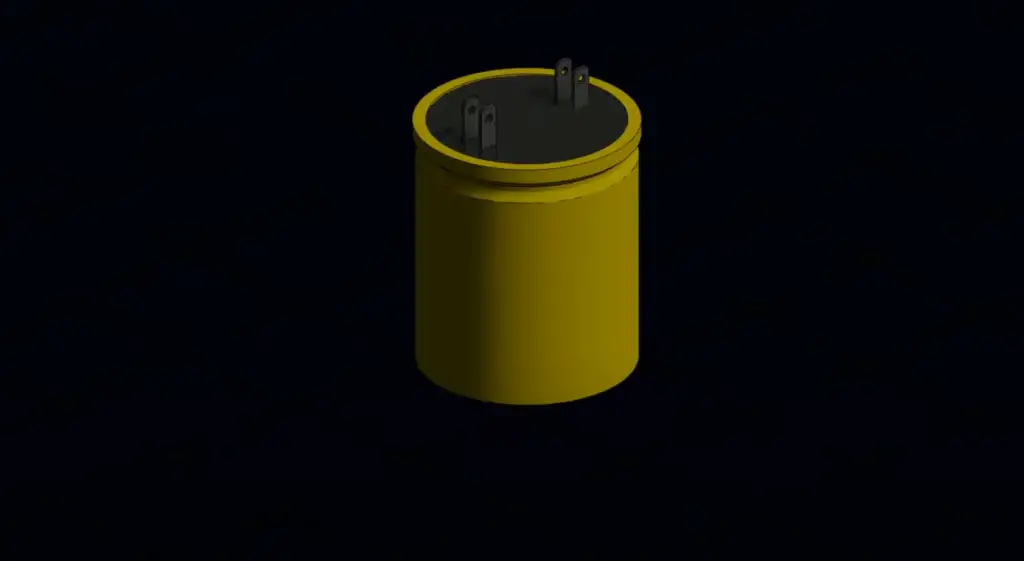### Capacitive Reactance

In a capacitive circuit, the flow of current is influenced by the capacitive reactance. Capacitive reactance, denoted by Xc, is the opposition encountered by the alternating current (AC) when passing through a capacitor. It is inversely proportional to the frequency of the AC signal and the capacitance of the capacitor .

Mathematically, the capacitive reactance can be calculated using the formula Xc = 1 / (2πfC), where f represents the frequency and C denotes the capacitance.

As the frequency increases, the capacitive reactance decreases, allowing more current to flow through the capacitor. Conversely, as the frequency decreases, the capacitive reactance increases, limiting the flow of current. This characteristic makes capacitors useful for filtering high-frequency signals and blocking direct current (DC).

### Capacitor Types

Capacitors come in various types, each with its unique characteristics and applications. Some common types of capacitors include:

• Electrolytic Capacitors: These capacitors are polarized and commonly used for high capacitance applications. They have a higher voltage rating and are suitable for power supply filtering and audio applications;
• Ceramic Capacitors: Ceramic capacitors are non-polarized and available in a wide range of capacitance values. They are compact, affordable, and find applications in decoupling, bypassing, and timing circuits;
• Film Capacitors: Film capacitors are non-polarized and offer excellent stability, high voltage ratings, and low leakage current. They are often used in high-frequency applications, motor circuits, and audio systems;
• Tantalum Capacitors: Tantalum capacitors are polarized and known for their high capacitance per volume. They find applications in portable electronics, telecommunications, and medical devices;
• Variable Capacitors: These capacitors allow for manual adjustment of the capacitance value. They are commonly used in tuning circuits, radio receivers, and antennas;

### Capacitor Ratings

Capacitors are rated based on several parameters that define their performance characteristics. The most important capacitor ratings include:

• Capacitance (C): Capacitance is the measure of a capacitor’s ability to store electrical charge and is measured in farads (F). Capacitors with higher capacitance can store more charge;
• Voltage Rating: The voltage rating indicates the maximum voltage a capacitor can handle without the risk of failure. It is crucial to choose a capacitor with a voltage rating higher than the maximum voltage it will encounter in the circuit;
• Tolerance: Tolerance specifies the allowable deviation in capacitance from the stated value. It is usually expressed as a percentage;
• Temperature Coefficient: The temperature coefficient denotes how a capacitor’s capacitance changes with temperature variations. It is important to consider this rating when capacitors are used in environments with extreme temperature fluctuations;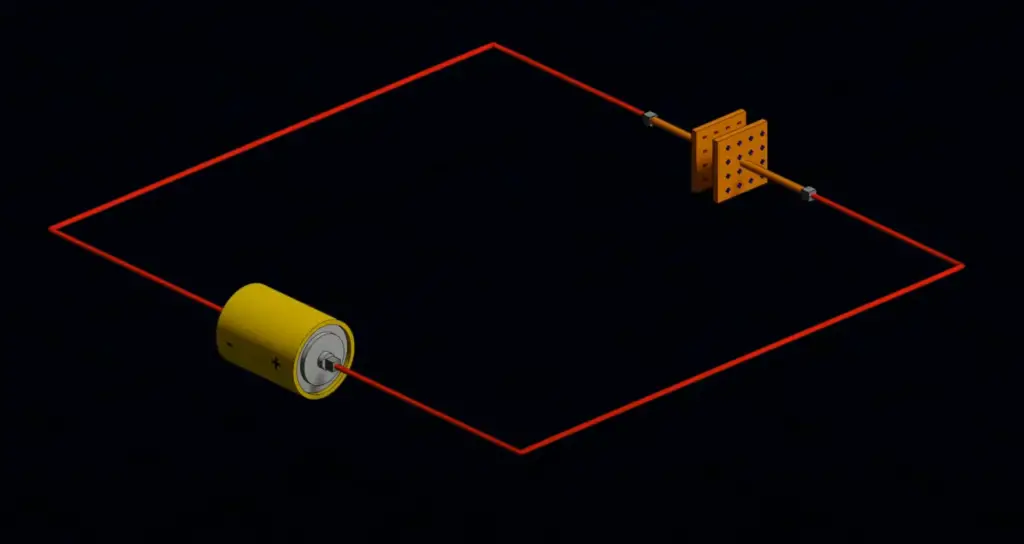### Capacitor Technologies

Capacitor technologies have evolved over time, leading to the development of capacitors with improved performance and reliability. Some notable capacitor technologies include:

• Aluminum Electrolytic Capacitors: These capacitors use an aluminum oxide film as the dielectric and electrolyte to achieve high capacitance values. They are commonly used in power supply applications;
• Multilayer Ceramic Capacitors (MLCCs): MLCCs consist of multiple ceramic layers, each with electrodes, stacked together. They offer high capacitance in small packages and are widely used in electronic devices;
• Solid Tantalum Capacitors: Solid tantalum capacitors utilize tantalum pentoxide as the dielectric material, providing high volumetric efficiency and stable capacitance. They are often used in portable electronic devices;
• Supercapacitors: Supercapacitors, also known as ultracapacitors, have extremely high capacitance values and can store and deliver energy rapidly. They find applications in hybrid vehicles, renewable energy systems, and power backup systems ;

## Explaining The Capacitor Theory:

### How Is a Capacitor Made?

A capacitor is essentially two conductive plates separated by an insulating material, called a dielectric. The conductive plates are usually made of thin metal foils that are wound or stacked together, while the dielectric can be anything from plastic to air. The choice of dielectric is important, as it determines the capacitor’s properties such as capacitance, voltage rating, and temperature stability.

One popular type of capacitor is the electrolytic capacitor, which has a liquid electrolyte that acts as its dielectric. These capacitors often have a larger capacitance rating and can handle higher voltages than other types. Another type is the ceramic capacitor, which uses a ceramic material as its dielectric and is known for its stability and small size.

### How Does a Capacitor Work?

Now that we know what a capacitor is and how it’s made, let’s move on to how it actually works. In essence, a capacitor stores electric charge and releases it when needed. When a voltage is applied to a capacitor, the electrons in one plate accumulate and repel electrons in the other plate, which causes a transfer of charge between the two plates. This creates an electric field in the capacitor and charges it up.

When the voltage source is removed, the capacitor will maintain its charge for a certain period of time, depending on its capacitance and the resistance of the circuit. This is because the capacitor’s dielectric doesn’t allow current to flow through it, which prevents the charge from dissipating. Once the capacitor is fully charged, it acts like an open circuit, effectively blocking any more current from flowing through it.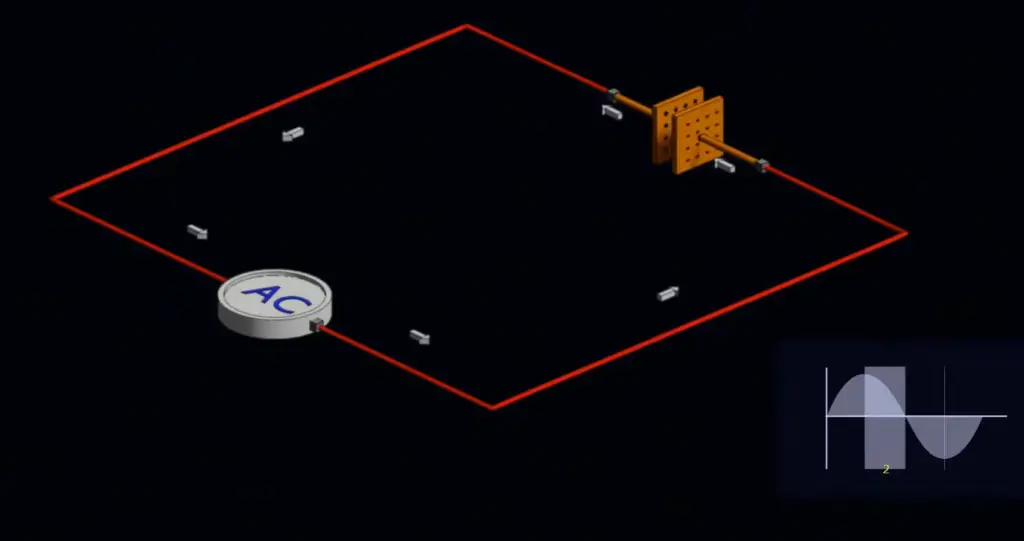### Charging and Discharging A Capacitor

Now that we know how a capacitor works, it’s important to understand the process of charging and discharging it. To charge a capacitor, you simply connect it across a voltage source, such as a battery or power supply.

As the voltage builds up across the capacitor, the current flowing into it decreases until it reaches zero once the capacitor is fully charged. The amount of time it takes to charge a capacitor depends on its capacitance and the resistance of the circuit it’s connected to.

To discharge a capacitor, you simply remove the voltage source and connect a load, such as a resistor, across its terminals. This allows the stored charge to flow out of the capacitor and through the load, creating a current. The rate at which the charge is dissipated depends on the capacitance of the capacitor and the resistance of the load.

### Calculating Charge and Voltage Of A Capacitor

We can calculate the charge and voltage of a capacitor using the following equations:

#### Q = CV

Where Q is the charge stored in the capacitor in coulombs, C is the capacitance in farads, and V is the voltage across the capacitor in volts.

#### V = Q/C

Where V is the voltage across the capacitor in volts, Q is the charge stored in the capacitor in coulombs, and C is the capacitance in farads.

These equations tell us that the amount of charge stored in a capacitor is directly proportional to its capacitance and the voltage applied to it. Similarly, the voltage across the capacitor is inversely proportional to its capacitance and the amount of charge stored in it .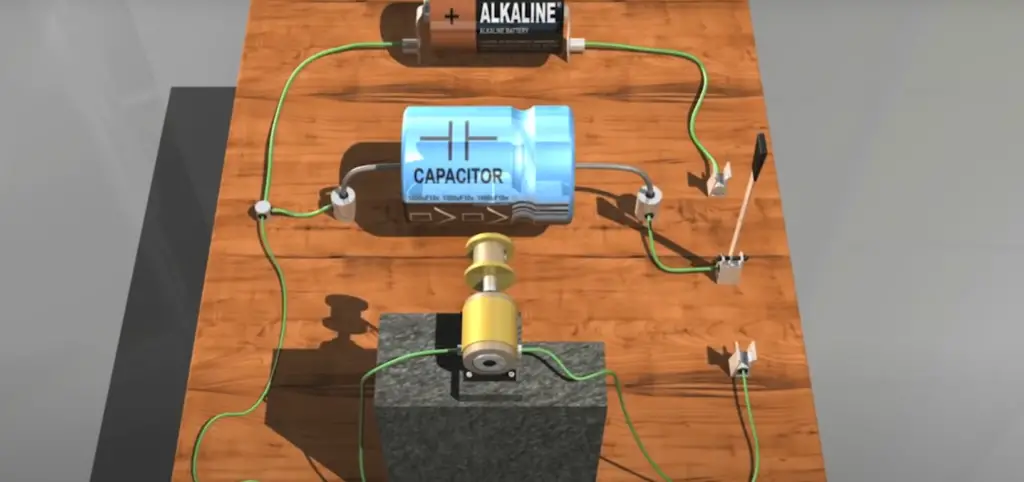### Calculating The Current Of A Capacitor

We can also calculate the current flowing through a capacitor using the following equation:

I = C * dV/dt

Where I is the current flowing through the capacitor in amperes, C is the capacitance in farads, and dV/dt is the rate of change of the voltage across the capacitor in volts per second.

This equation tells us that the current flowing through a capacitor is directly proportional to its capacitance and the rate of change of the voltage across it. We can use this equation to determine the behavior of a capacitor in an AC circuit, where the voltage is constantly changing.

### Summing Up – Does Current Flow Through A Capacitor?

Yes, current does flow through a capacitor, but not in the same sense as it flows through a conductor, as a capacitor is designed to store and release electric charge.

When a voltage is applied across the terminals of a capacitor, an electric field develops across the dielectric, causing a net positive charge to collect on one plate and net negative charge to collect on the other plate.

As a result, the capacitor is charged, which means that there is flow of charge through the source circuit . If a time-varying voltage is applied across the leads of the capacitor, the source experiences an ongoing current due to the charging and discharging cycles of the capacitor. However, no current actually flows through the dielectric itself.

The capacitance of a conductor is a measure of its ability to store charge and is dependent on factors such as the distance between the conductive plates and the dielectric material used.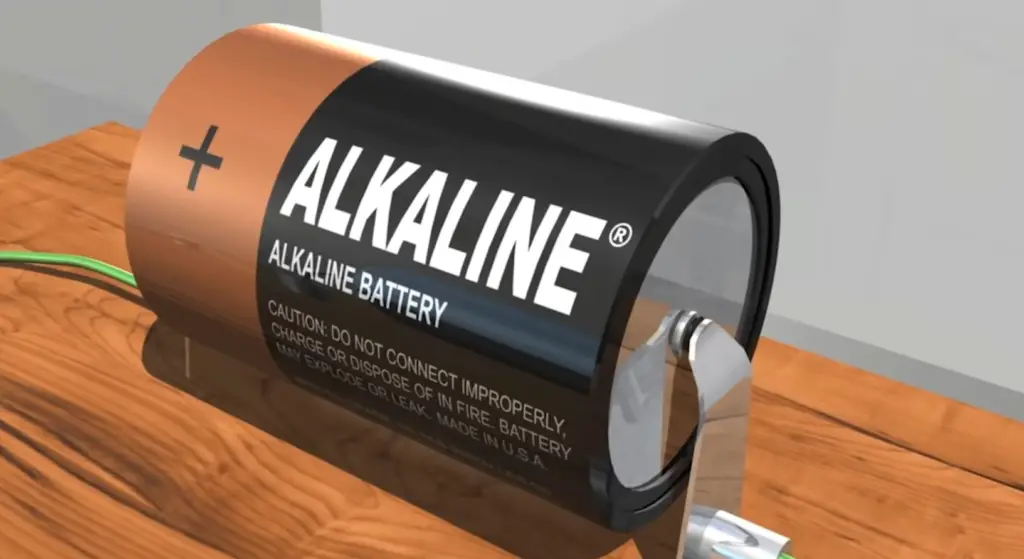## FAQ:

### 1. What is the current flowing in a capacitor called?

The current flowing in a capacitor is called the charging or discharging current. When a capacitor is connected to a voltage source, it charges and discharges, causing a flow of electric current.

### 2. Is current through a capacitor 0?

No, the current through a capacitor is not always zero. Initially, when a capacitor is uncharged and connected to a voltage source, the current is maximum as the capacitor charges up. As the charging progresses, the current gradually decreases until it reaches zero once the capacitor is fully charged. Similarly, during discharging, the current starts at a maximum value and decreases until it reaches zero.

### 3. Does current flow when a capacitor is full?

Once a capacitor is fully charged, the current flow stops. This is because the capacitor has reached its maximum charge, and no further charge can be stored. In an ideal capacitor, there is no leakage, so the current ceases to flow.

### 4. What happens to current when the capacitor is charged?

When a capacitor is charging, the current gradually decreases over time. Initially, the current is high, as the capacitor acts like a short circuit, allowing the flow of current to build up the charge on its plates. However, as the capacitor charges and the voltage across it increases, the potential difference between the capacitor and the source decreases, resulting in a decrease in current flow.

### 5. Does current drop across a capacitor?

Yes, the current drops across a capacitor. In an ideal capacitor, the current through it is directly proportional to the rate of change of voltage across it . As the voltage across the capacitor increases, the current decreases, and vice versa.

### 6. What two factors determine the capacitive reactance of a capacitor?

The capacitive reactance of a capacitor is determined by two factors: the frequency of the AC signal passing through the capacitor and the capacitance of the capacitor itself. The capacitive reactance (Xc) can be calculated using the formula Xc = 1 / (2πfC), where f represents the frequency and C represents the capacitance.

### 7. What is the capacitive reactance of the capacitor?

The capacitive reactance (Xc) of a capacitor is the opposition encountered by an alternating current (AC) when passing through a capacitor. It is inversely proportional to the frequency of the AC signal and the capacitance of the capacitor. The formula to calculate capacitive reactance is Xc = 1 / (2πfC), where Xc represents the capacitive reactance, f represents the frequency, and C represents the capacitance .

### 8. How does current flow through a capacitor in an AC circuit?

In an AC circuit, the current flow through a capacitor alternates in direction due to the alternating voltage source. During the positive half-cycle of the AC signal, the capacitor charges, allowing current to flow from the source to the capacitor.

In the negative half-cycle, the capacitor discharges, releasing the stored charge and allowing current to flow back to the source. This alternating current flow through the capacitor results in a phase shift between the voltage and current waveforms.

### 9. Why does current stop flowing through a capacitor?

Current stops flowing through a capacitor when it becomes fully charged or discharged. Once the capacitor reaches its maximum charge, it cannot store any additional charge, and the current flow ceases. In an ideal capacitor, with no leakage, the current stops flowing completely.

### 10. Does current flow through a fully charged capacitor?

No, current does not flow through a fully charged capacitor in an ideal situation. Once a capacitor reaches its maximum charge, it cannot store any more charge, and the current flow stops. However, in practical situations, there might be a small amount of leakage current due to the imperfections of real capacitors.

### 11. Does DC current flow through a capacitor?

No, a capacitor blocks the flow of direct current (DC) in an ideal situation. When a DC source is connected to a capacitor, initially, there is a surge of current as the capacitor charges up. However, once the capacitor is fully charged, it acts as an open circuit and blocks the flow of DC current.

### 12. How does a capacitor release energy?

A capacitor releases energy when it discharges. During the charging process, the capacitor stores electrical energy in its electric field. When the circuit is connected to a load or a discharge path, the capacitor begins to release the stored energy. The stored energy is converted back into electrical current, providing power to the load or circuit. The rate at which the capacitor releases energy depends on the discharge path and the capacitance of the capacitor.